output.to from Sideway
Draft for Information Only

# Content

``` Body Force of 3D Body   Center of Gravity of a 3D Body  Center of Mass  Centroid of Volume```

# Body Force of 3D Body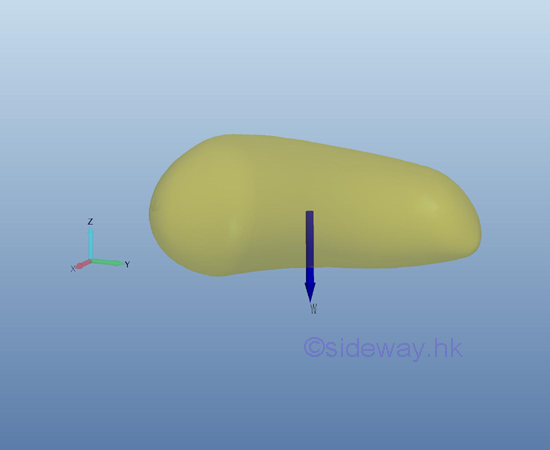The weight of the body is equal to the force exerted by the earth on the body. Imply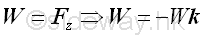The negative sign indicates the negative sense of the force relative to the coordinate system.

## Center of Gravity of a 3D Body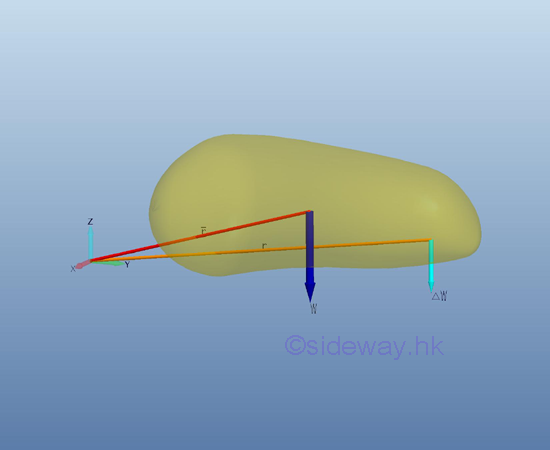The force of gravity acts on each of the particles of the entire body. By dividing the body into small finite regions or elements, the weight of the body can also be divided into small forces distributing over the 3D body. For a 3D body of n element, the weight of the 3D body is equal to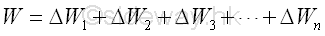As in the equivalent systems of forces, two systems of forces are equivalent at the point of interest, if: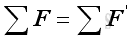and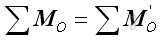By assuming the forces exerted by the earth on the elements of 3D body are parallel, the resultant force is equal to a single force in the same direction. Therefore, the magnitude of the body force of the 3D body is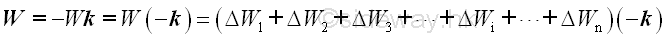By denoting the position vector of the element of the 3D body relative to the origin of coordinate system as ri, the position vector r of the center of gravity of the 3D body where the resultant force W applied can be determined by equating the moment of the resultant force W about the origin of coordinate system and the corresponding mements of elements of the 3D body about the origin of coordinate system. Imply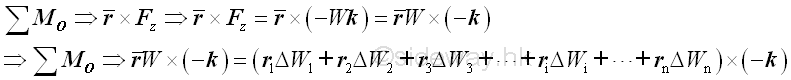Therefore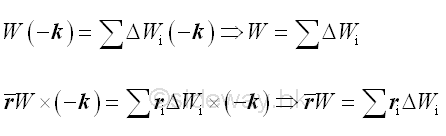When the number of elements n is increaed toward infinity by taking the limit as the size of each element approaches zero, Imply,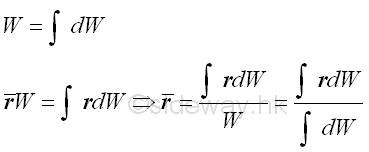Since the center of gravity of the 3D body is a function of the position vector and the elemental weight, and the origin of coordinate system is an arbitrary coordinate system, the orientation of the 3D body does not affect the position vector of the center of gravity of the 3D body.

In other words, the position vector of the elemental weight can be considered as weighted position vector. Therefore, in general, through vector decomposition, the position vectors r and  r can be resolved into three rectangular componts, Imply the  scalar coordinates x , y and z of the center of gravity of the 3D body is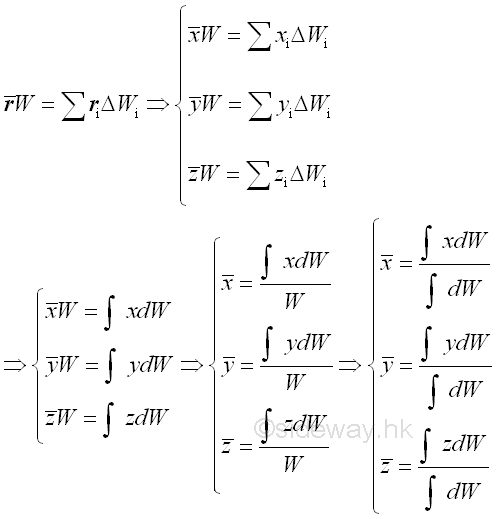## Center of Mass

The weight of an object is the gravitational force exerted by the earth on the body and can be defined as the mass of the object times the acceleration due to gravity between the object and the earth. Mass is the fundamental property of an object and is defined as the numerical measure of the amount of inertia matter of the object. Although, in general, the gravitational force field of earth is not uniform. For example, the gravitational force on an artificial satellite is not uniform. But for practical static mechanical problems, the gravitational force of earth acting on an object is usually assumed to be uniform at all places. For an uniform gravitational force field,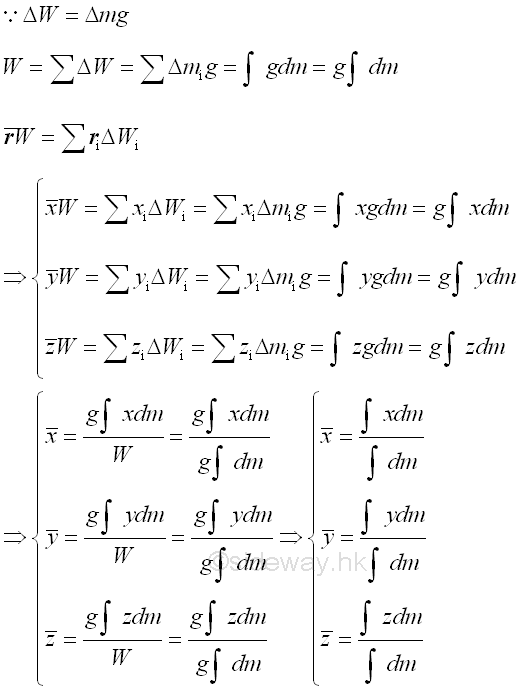Therefore, the center of mass is same as the center of gravity in an uniform gravitational force field.

## Centroid of Volume

The weight of an object can also be expressed in terms of density, ρ. For a homogenous three dimensional body, the weight of an element of the plane body of voulume, ΔV can be expressed as,Therefore, the centroid of volume of a homogeneous three dimensional body in an uniform gravitational force field is same as the center of gravity or center of mass.

ID: 120600009 Last Updated: 6/13/2012 Revision: 0 Ref:References

1. I.C. Jong; B.G. rogers, 1991, Engineering Mechanics: Statics and Dynamics
2. F.P. Beer; E.R. Johnston,Jr.; E.R. Eisenberg, 2004, Vector Mechanics for Engineers: StaticsHome 5

Management

HBR 3

Information

Recreation

Culture

Chinese 1097

English 339

Computer

Hardware 249

Software

Application 213

Latex 52

Manim 205

KB 1

Numeric 19

Programming

Web 289

Unicode 504

HTML 66

CSS 65

SVG 46

ASP.NET 270

OS 429

Python 72

Knowledge

Mathematics

Algebra 84

Geometry 34

Calculus 67

Engineering

Mechanical

Rigid Bodies

Statics 92

Dynamics 37

Control

Natural Sciences

Electric 27